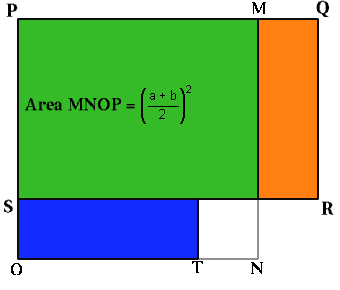#### You may also like### Diophantine N-tuples

Can you explain why a sequence of operations always gives you perfect squares?### DOTS Division

Take any pair of two digit numbers x=ab and y=cd where, without loss of generality, ab > cd . Form two 4 digit numbers r=abcd and s=cdab and calculate: {r^2 - s^2} /{x^2 - y^2}.### Sixational

The nth term of a sequence is given by the formula n^3 + 11n . Find the first four terms of the sequence given by this formula and the first term of the sequence which is bigger than one million. Prove that all terms of the sequence are divisible by 6.

# AMGM

##### Age 14 to 16Challenge LevelIf we choose any two numbers, call them $a$ and $b$ $(b < a)$ and work out the arithmetic mean $(a+b)/2$ and the geometric mean $\sqrt(ab)$ then the arithmetic mean is always bigger than the geometric mean. How can we prove it? One way is to use this diagram. Clement Goh, age 12 years, from River Valley High, Singapore sent a good solution.

In the diagram the measurements are:
PQ = a
PS = b
PM = PO = (a + b)/2
MQ = SO = TN = (a - b)/2

The blue rectangle measures (a - b)/2 by b , the same as the orange rectangle.

Rectangle PQRS, with area ab, is made up of the green plus the orange rectangle..
The square MNOP, with area [ (a + b)/2 ] 2 , is made up of the green plus the blue rectangles plus the yellow square.

area of MNOP - area PQRS = the area of the yellow square = [ (a - b)/2 ] 2 .

Hence the area of PQRS < area MNOP, that is ab < [ (a + b)/2 ] 2 .

Taking square roots this shows that the geometric mean $\sqrt(ab)$ is less than the arithmetic mean $[(a + b)/2]$.